Courses

# Ray And Wave Optics MCQ Level - 1

## 30 Questions MCQ Test Exclusive Video Lectures of Class 12 Physics by Experts | Ray And Wave Optics MCQ Level - 1

Description
This mock test of Ray And Wave Optics MCQ Level - 1 for Class 12 helps you for every Class 12 entrance exam. This contains 30 Multiple Choice Questions for Class 12 Ray And Wave Optics MCQ Level - 1 (mcq) to study with solutions a complete question bank. The solved questions answers in this Ray And Wave Optics MCQ Level - 1 quiz give you a good mix of easy questions and tough questions. Class 12 students definitely take this Ray And Wave Optics MCQ Level - 1 exercise for a better result in the exam. You can find other Ray And Wave Optics MCQ Level - 1 extra questions, long questions & short questions for Class 12 on EduRev as well by searching above.
QUESTION: 1

Solution:
QUESTION: 2

Solution:
QUESTION: 3

### Consider the situation shown in Fig. Water (μ = 4/3) isfilled in a beaker upto a height of 10 cm.A plane mirror is fixed at a height of 5 cm from thesurface of water. Distance of image from the mirror afterreflection from it of an object O at the bottom of the beaker is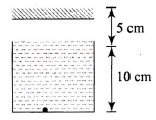Solution:
QUESTION: 4

Refraction takes place at a concave spherical boundaryseparating glass-air medium. For the image to be real,the object distance (μg = 3/2)

Solution:
QUESTION: 5

The refractive index of a prism is 2. The prism canhave a maximum refracting angle of

Solution:
QUESTION: 6

Angle of minimum deviation is equal to the angle ofprism of an equilateral glass prism. The angle ofincidence at which minimum deviation will be obtained is

Solution:
QUESTION: 7

A plano-convex lens fits exactly into a plano-concavelens. Their plane surfaces are parallel to each other. Ifthe lenses are made of different material of refractiveindices μ1 and μand R is the radius of curvature of the curved surface of the lenses, then focal length of thecombination is

Solution:
QUESTION: 8

A parallel beam of light is incident on the system oftwo convex lenses of focal lengths f1 = 20 cm and f2 = 10 cm. What should be the distance between thetwo lenses so that rays after refraction from both thelenses pass undeviated?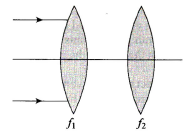Solution:
QUESTION: 9

A plano-convex glass lens (μg = 3/2) of radius ofcurvature R = 10 cm is placed at a distance of ‘b’ froma concave lens of focal length 20 cm.What should be the distance ‘a’ of a point object Ofrom the plano-convex lens so that the position of finalimage is independent of’b’?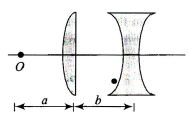Solution:
QUESTION: 10

A point object is placed at a distance of 25 cm from aconvex lens of focal length 20 cm. If a glass slab ofthickness t and refractive index 1.5 is inserted between the lens and the object, the image is formed at infinity. The thickness t is

Solution:
QUESTION: 11

A convex lens of focal length 10 cm is painted black atthe middle portion as shown in Fig. An object is placedat a distance of 20 cm from the lens.Then,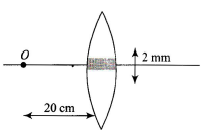Solution:
QUESTION: 12

Consider an equiconvex lens of radius of curvature Rand focal length f. If f > R, the refractive index μ of thematerial of the lens

Solution:
QUESTION: 13

An equiconvex lens is cut into two halves along (i) XOX’ and (ii) YOY” as shown in Fig. Let f, f ' ,f '' be the focal length of the lens, of each half in case (i), and of each half in case (ii), respectively, Choose the correct statement from the following: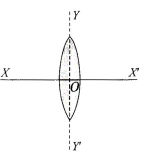Solution:
QUESTION: 14

A spherical mirror forms an image of magnification 3.The object distance, if focal length of mirror is 24 cm,may be

Solution:
QUESTION: 15

The critical angle for light going from medium X intomedium Y is θ . The speed of light in medium X is v.The speed of light in medium Y is

Solution:
QUESTION: 16

A convex lens of focal length 1.0 m and a concave lens of focal length 0.25 m are 0.75 m apart. A parallel beam of light is incident on the convex lens. The beam emerging after refraction from both lenses is

Solution:
QUESTION: 17

A convex lens A of focal length 20 cm and a concavelens G of focal length 5 cm are kept along the sameaxis with the distance d between them. If a parallelbeam of light falling on A leaves B as a parallel beam,then distance d in cm will be

Solution:
QUESTION: 18

A convex lens forms an image of an object placed 20cm away from it at a distance of 20 cm on the otherside of the lens. If the object is moves 5 cm toward thelens, the image will be

Solution:
QUESTION: 19

With a concave mirror, an object is placed at a distance x1 from the principal focus, on the principal axis. The image is formed at a distance x2 from the principal focus. The focal length of the mirror is

Solution:
QUESTION: 20

A concave mirror is placed on a horizontal table withits axis directed vertically upward. Let O be the pole ofthe mirror and C its center of curvature. A point objectis placed at C. It has a real image, also located at C. Ifthe mirror is now filled with water, the image will be

Solution:
QUESTION: 21

A point source of light B is placed at a distance L infront of the center of a mirror of width d hung verticallyon a wall. A man walks in front of the mirror along a lineparallel to the mirror at a distance 2L from it as shownin Fig.the greatest distance over which he can see theimage of the light source in the mirror is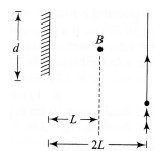Solution:
QUESTION: 22

When an object is kept at a distance of 30 cm from aconcave mirror, the image is formed at a distance of10 cm. If the object is moved with a speed of 9 cm s-1 the speed with which the image moves is

Solution:
QUESTION: 23

A convex mirror of radius of curvature 1.6 m has an object placed at a distance of 1 m from it. The image is formed at a distance of

Solution:
QUESTION: 24

In the above question, the magnification is

Solution:
QUESTION: 25

A convex mirror and a concave mirror of radius 10 cmeach are placed 15 cm apart facing each other. Anobject is placed midway between them. If the reflectionfirst takes place in the concave mirror and then inconvex mirror, the position of the final image is

Solution:
QUESTION: 26

A small piece of wire bent into an L shape, with uprightand horizontal portions of equal lengths, is placed withthe horizontal portion along the axis of the concavemirror whose radius of curvature is 10 cm. If the bendis 20 cm from the pole of the mirror, then the ratio ofthe lengths of the images of the upright and horizontalportions of the wire is

Solution:
QUESTION: 27

The image of an object placed on the principal axis ofa concave mirror of focal length 12 cm is formed at apoint which is 10 cm more distance from the mirror than the object. The magnification of the image is

Solution:
QUESTION: 28

A clear transparent glass sphere (μ =1.5) of radius R is immersed in a liquid of refractive index 1.25. A parallel beam of light incident on it will converge to a point. The distance of this point from the center will be

Solution:
QUESTION: 29

A ray of light traveling in glass (μ = 3/2) is incident ona horizontal glass-air surface at the critical angle θC Ifa thin layer of water (μ = 4/3) is now poured on theglass-air surface, the angle at which the ray emergesinto air at the water-air surface is

Solution:
QUESTION: 30

A ray of light enters a rectangular glass slab of refractiveindex√3 at an angle of incidence 60°. It travels adistance of 5 cm inside the slab and’ emerges out ofthe slab. The perpendicular distance between theincident and the emergent rays is

Solution: